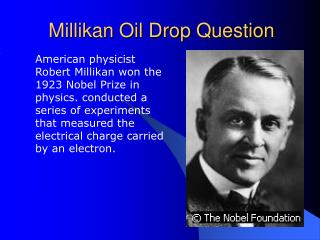DownloadDownload PresentationMillikan Oil Drop Question

# Millikan Oil Drop Question

Download Presentation## Millikan Oil Drop Question

- - - - - - - - - - - - - - - - - - - - - - - - - - - E N D - - - - - - - - - - - - - - - - - - - - - - - - - - -
##### Presentation Transcript

1. Millikan Oil Drop Question • American physicist Robert Millikan won the 1923 Nobel Prize in physics. conducted a series of experiments that measured the electrical charge carried by an electron.

2. Problem • Two large parallel metal plates are separated by a distance of 2.0 x 10-3 meters. • The plates are attached to a 4.0 x 103 volt source. + + + + + + + + + + - - - - - - - - - - -

3. Calculate • The FIELD INTENSITY between the plates ( E = V/d) • Determine the FORCE on an electron in the FIELD ( F = q E) • Calculate the WORK necessary to move an electron from the positive plate to the negative plate in ELECTRON VOLTS and in Joules ( W = q V)

4. + + + + + + + + + + Sample Solutions Field intensity - - - - - - - - - - - • E = V / d = (4.0 x 10 3 V) / ( 2.0 x 10-3 m) = 2.0 x 10 6 V/m Two large parallel metal plates are separated by a distance of 2.0 x 10-3 m. The plates are attached to a 4.0 x 10 3 V

5. Force Example Calculate the force exerted on an electron on an electric field, whose intensity is 2.0 x 10 –19 C • F = q E = ( 1.6 x 10 –19 C) (2.0 x 10 3 N/C) = 3.2 x 10 –16 N

6. Work Example Calculate the work necessary to move an electron from the positive plate to the negative plate in eV and in J. • W = qV = 1 electron x (4.0 x 10 3 V) = 4.0 x 10 3 eV Since : 1 eV = 1.6 x 10 –19 J W = 1 electron x (4.0 x 10 3 V) = (1.6 x 10 –19 J/ev)(4.0 x 10 3 eV) = 6.4 x 10 –16 J

7. Summary • Robert Millikan conducted a series of experiments that measured the electrical charge carried by an electron. • Using the potential ( volts ) formulas based on an electron ‘s “pressure” • Solve for FIELD INTENSITY, FORCE, and WORK on the electron.